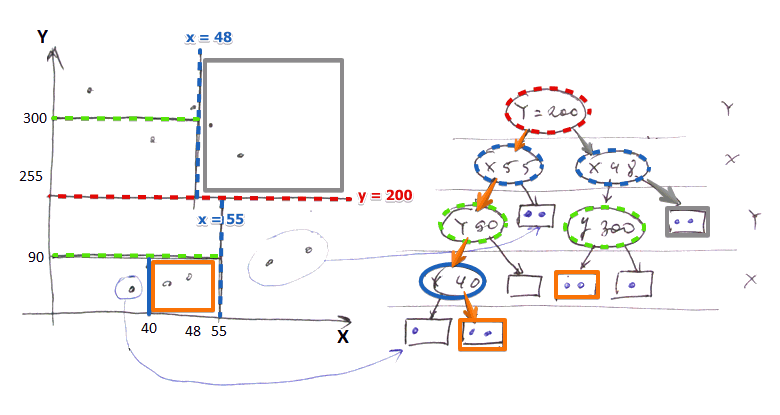# ML Wiki

## Classical $kd$-Tree• split attributes at different levels are different
• in the example: first split by $Y$, then by $X$, then by $Y$...
• interior nodes of the tree contain
• attribute on which we divided the space
• a dividing value
• left and right pointers (only 2!)
• leaves are blocks with records

## Operations

### Lookup

• similar to binary search trees
• but need to use only attribute specified in a interior node of the tree

### Insertion

• look up the block
• if there's room - put it there
• not - split into 2
• we divide by the most appropriate attribute
• and create a new interior node that points to the split halves

## Queries

Good for See Multi-Dimensional Indexes#Typical Queries

• Point Queries (just lookup)
• Partial Match
• suppose we specified only $Y$
• then if it's not $Y$ follow both left and right pointers
• if it's $Y$ then use the dividing value in the node to decide whether to go left or right

Reasonable Support

• Nearest-Neighbor

The described algorithm is for Main Memory, not for disk

• Multi-Way Branches
• like in B-Tree: $n$ keys, $n + 1$ pointers
• hard to merge to keep it balanced
• Several Nodes Per Block
• Keep 2 children per block as described
• but store several nodes per one block
• to minimize I/O it's better to put in the same block records that are likely to be accessed together
• For example, a node and it's descendants